# MA.912.AR.8.1Export Print
Write and solve one-variable rational equations. Interpret solutions as viable in terms of the context and identify any extraneous solutions.

### Clarifications

Clarification 1: Within the Algebra 2 course, numerators and denominators are limited to linear and quadratic expressions.
General Information
Subject Area: Mathematics (B.E.S.T.)
Strand: Algebraic Reasoning
Status: State Board Approved

## Benchmark Instructional Guide

### Terms from the K-12 Glossary

• Linear expression
• Rational expression
• Rational number

### Vertical Alignment

Previous Benchmarks

Next Benchmarks

### Purpose and Instructional Strategies

In Algebra I, students solved one variable equations including linear, quadratic and absolute value, and determined if the solution(s) was viable. In Math for College Algebra, students solve one variable equations involving rational expressions.
• Instruction includes making the connection to operations with fractions (MTR.5.1)
• For example, when solving rational equations that involve addition or subtraction, students can find common denominators to assist in solving.
• Given the equation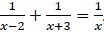, students can determine a common denominator of ($x$ − 2)($x$ + 3) to rewrite the equation as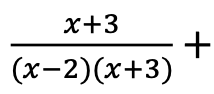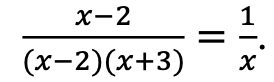. Students should recognize that since the fractions on the ($x$ − 2)($x$ + 3) left side of the equation have the same denominator, the equation can be rewritten as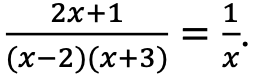From here students can either solve using proportional reasoning (MA.7.AR.3) or can find common denominators between fractions on either side of the equal side and then set the numerators equivalent to one another.
• Instruction includes how to determine what the solutions to the equation are and whether they are viable (MTR.6.1). Students should have experience with rational equations that produce different types of solutions: more than one, exactly one, and extraneous.
• Instruction provides opportunities for students to discuss why extraneous solutions may arise with rational equations (MTR.4.1)
• Instruction gives students the opportunity to discuss constraints and what effect those constraints have on the solution(s) to the equations (MTR.4.1).

### Common Misconceptions or Errors

• Students may struggle to determine if a common denominator is needed to solve a rational equation.
• If students determine a common denominator is needed, they may struggle to decide what factors are needed to be multiplied to the numerator and the denominator while keeping the rational equation equivalent.
• Students may forget to test the solutions in the original equation to determine if it is extraneous or not.

• Explain how you could find the solution(s) to the rational equation given. Share your strategy with a partner.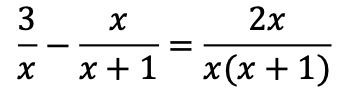(MTR.5.1)
• Create a rational equation with the following:
• a. One solution
• b. Two solutions
• c. Infinitely many solutions
• d. An extraneous solutions

• At a local high school, sophomores are designing and printing school lanyards for the upcoming freshman class. An online printing company charges \$40 set up fee and \$2.30 for each printed lanyard.
• Part A. Create an equation to represent the average cost $C$($x$), in dollars, per lanyard if $l$ lanyards are printed using this company.
• Part B. What is the average cost per lanyard to print 55 lanyards? 105 lanyards?
• Part C. How many lanyards should be printed to have an average cost of \$2.50 or less per lanyard? Explain how you know.

### Instructional Items

Instructional Item 1
• Chase and his brother like to play basketball. About a month ago they decided to keep track of how many games they have each won. As of today, Chase has won 18 out of 30 games against his brother.
• Part A. How many games would Chase have to win in a row in order to have a 75% winning record?
• Part B. How many games would Chase have to win in a row in order to have a 90% winning record?
• Part C. Is Chase able to reach a 100% winning record? Explain why or why not.
• Part D. Suppose that after reaching a winning record of 90% in Part B, Chase had a losing streak. How many games in a row would Chase have to lose in order to drop down to a winning record below 55% again?

Instructional Item 2

• An equation is given below. Determine the solution(s), including any extraneous solutions.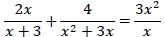*The strategies, tasks and items included in the B1G-M are examples and should not be considered comprehensive.

## Related Courses

This benchmark is part of these courses.
1200330: Algebra 2 (Specifically in versions: 2014 - 2015, 2015 - 2022, 2022 and beyond (current))
1200340: Algebra 2 Honors (Specifically in versions: 2014 - 2015, 2015 - 2022, 2022 and beyond (current))
1200400: Foundational Skills in Mathematics 9-12 (Specifically in versions: 2014 - 2015, 2015 - 2022, 2022 and beyond (current))
7912095: Access Algebra 2 (Specifically in versions: 2016 - 2018, 2018 - 2019, 2019 - 2022, 2022 and beyond (current))
1200710: Mathematics for College Algebra (Specifically in versions: 2022 and beyond (current))

## Related Access Points

Alternate version of this benchmark for students with significant cognitive disabilities.
MA.912.AR.8.AP.1: Solve one-variable rational equations and identify any extraneous solutions.

## Related Resources

Vetted resources educators can use to teach the concepts and skills in this benchmark.

## Lesson Plan

A Rational Representation:

Students will tackle a real-world situation regarding starting a business that requires a rational equation to evaluate the plan. Students will determine a method and set of steps for solving rational equations and then revisit the original scenario and solve using the new method they have synthesized. Students will also explore, through collaborative learning structures, the concept of extraneous solutions.

Type: Lesson Plan

## Original Student Tutorials

Solving Rational Equations: Using Common Denominators:

Learn how to solve rational functions by getting common denominators in this interactive tutorial.

Type: Original Student Tutorial

Solving Rational Equations: Cross Multiplying:

Learn how to solve rational linear and quadratic equations using cross multiplication in this interactive tutorial.

Type: Original Student Tutorial

## Original Student Tutorials Mathematics - Grades 9-12

Solving Rational Equations: Cross Multiplying:

Learn how to solve rational linear and quadratic equations using cross multiplication in this interactive tutorial.

Solving Rational Equations: Using Common Denominators:

Learn how to solve rational functions by getting common denominators in this interactive tutorial.

## Student Resources

Vetted resources students can use to learn the concepts and skills in this benchmark.

## Original Student Tutorials

Solving Rational Equations: Using Common Denominators:

Learn how to solve rational functions by getting common denominators in this interactive tutorial.

Type: Original Student Tutorial

Solving Rational Equations: Cross Multiplying:

Learn how to solve rational linear and quadratic equations using cross multiplication in this interactive tutorial.

Type: Original Student Tutorial

## Parent Resources

Vetted resources caregivers can use to help students learn the concepts and skills in this benchmark.